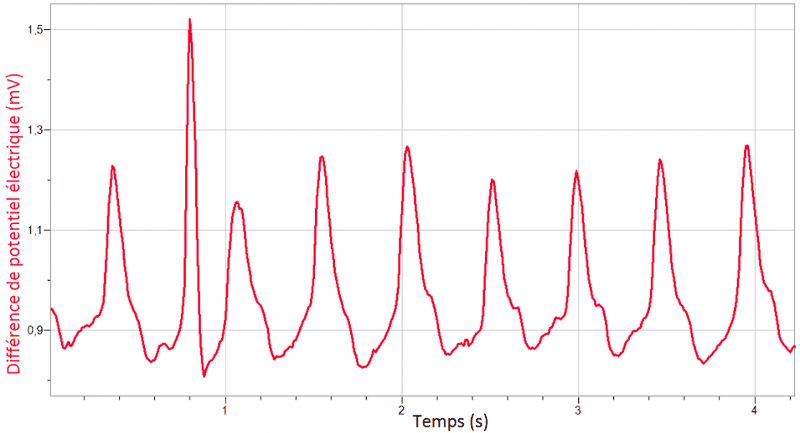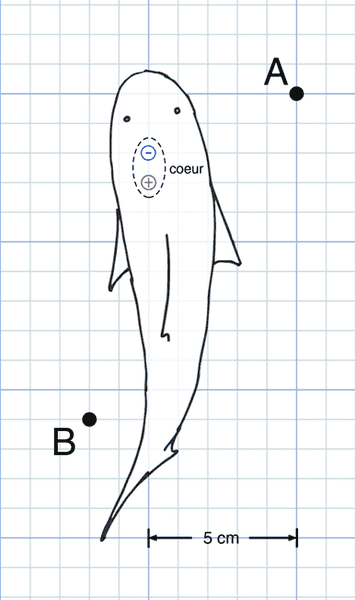# Electroreception, Electric field and charges

Hi guys. This is my question. I just wondering what equation that i'm suppose to use.

## Homework Statement

What is the maximum distance at which a shark can detect the electric signal emitted by the trout’s heart if the predator is at a point on an axis passing through the centre of the heart? Assume the axis is parallel to the x-axis.Figure 6: Electrical potential difference measured at Points A and B by the sensors shown in Figure 7. This is the beating of a brook trout’s heart recorded when the trout is in the position shown in Figure 7.Figure 7: Scale drawing of trout seen from above in the aquarium when the measurement is taken by the electrocardiograph. The positions of sensors A and B and the electric dipole representing the heart are all shown.
sensitivity threshold of the shark is up to 0.5 μV/m

## Homework Equations

E=ke(q/r^2) (the only equation that I can relate to)

## The Attempt at a Solution

This question is an assumption type question. If any of you guys know how to solve this or just any equation involve is a big help. I tried to read the theoretical part but still it confusing. i tried to use the above equation but i don't actually know how to find the charge of the dipole from the picture. Beside that, I'm wondering if the shark sensitivity is related to the distance or not.

Simon Bridge